Case Based Questions (MCQ)

Chapter 13 Class 12 Probability
Serial order wise

## Based on the above information answer the following questions: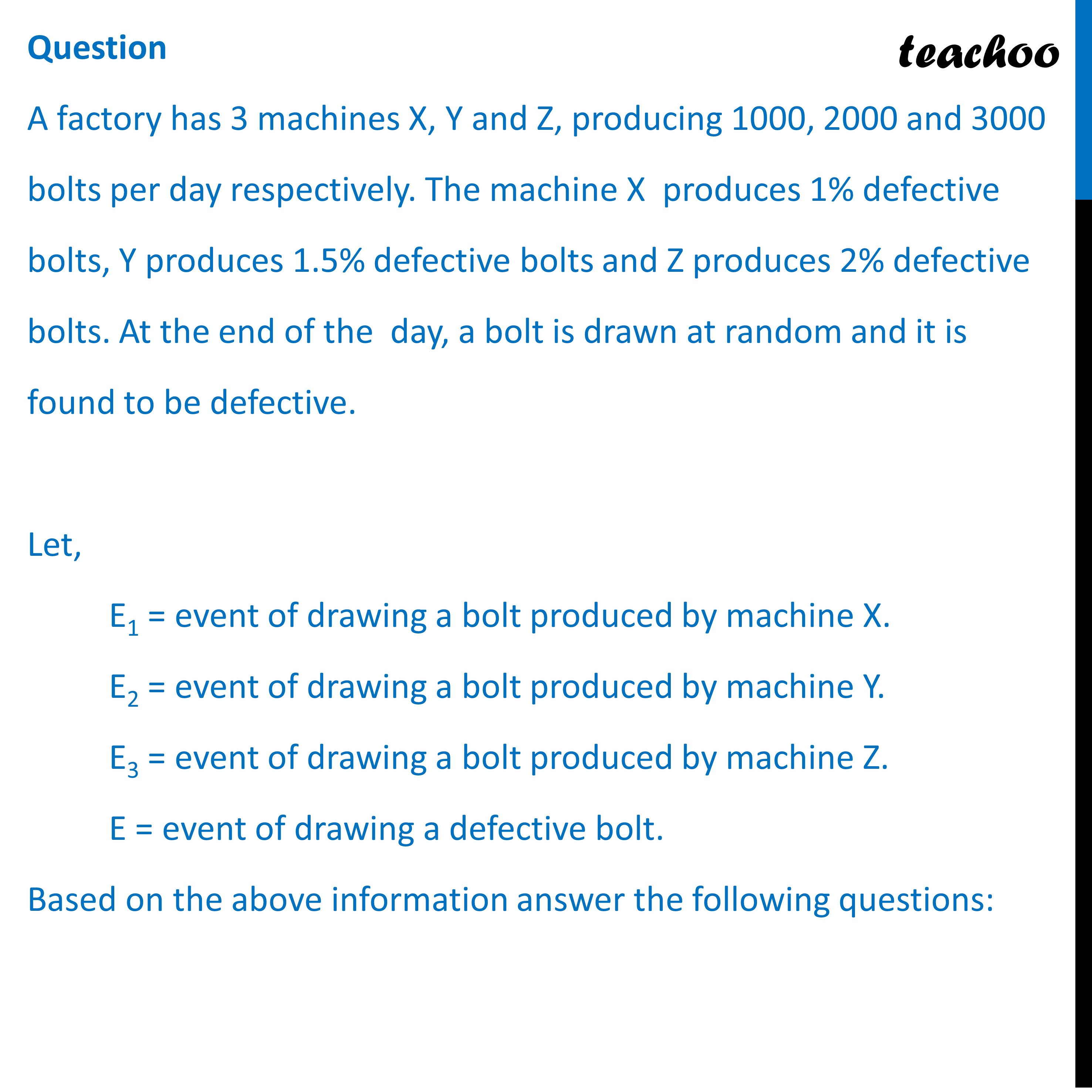## (D) 1/4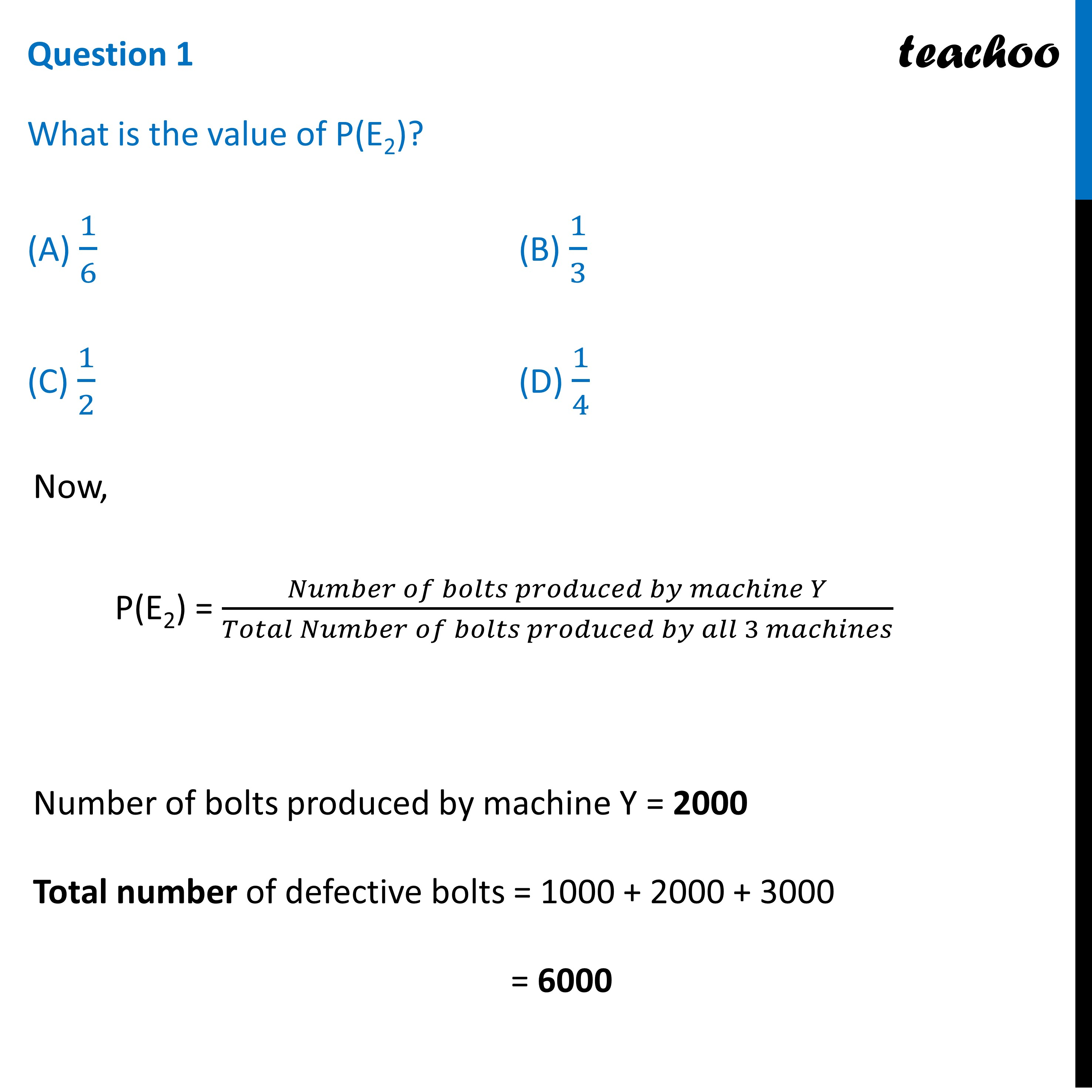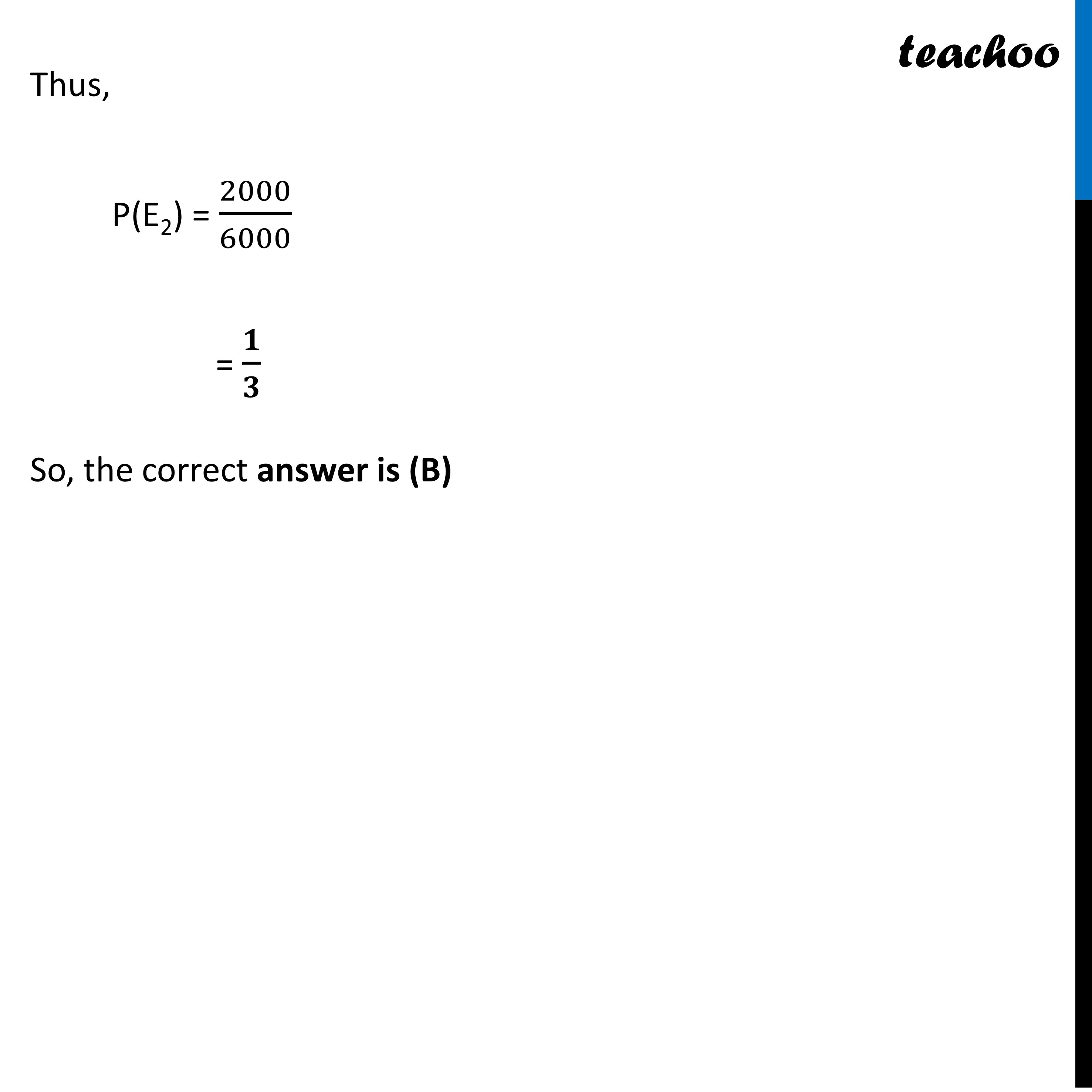## (D) 1/10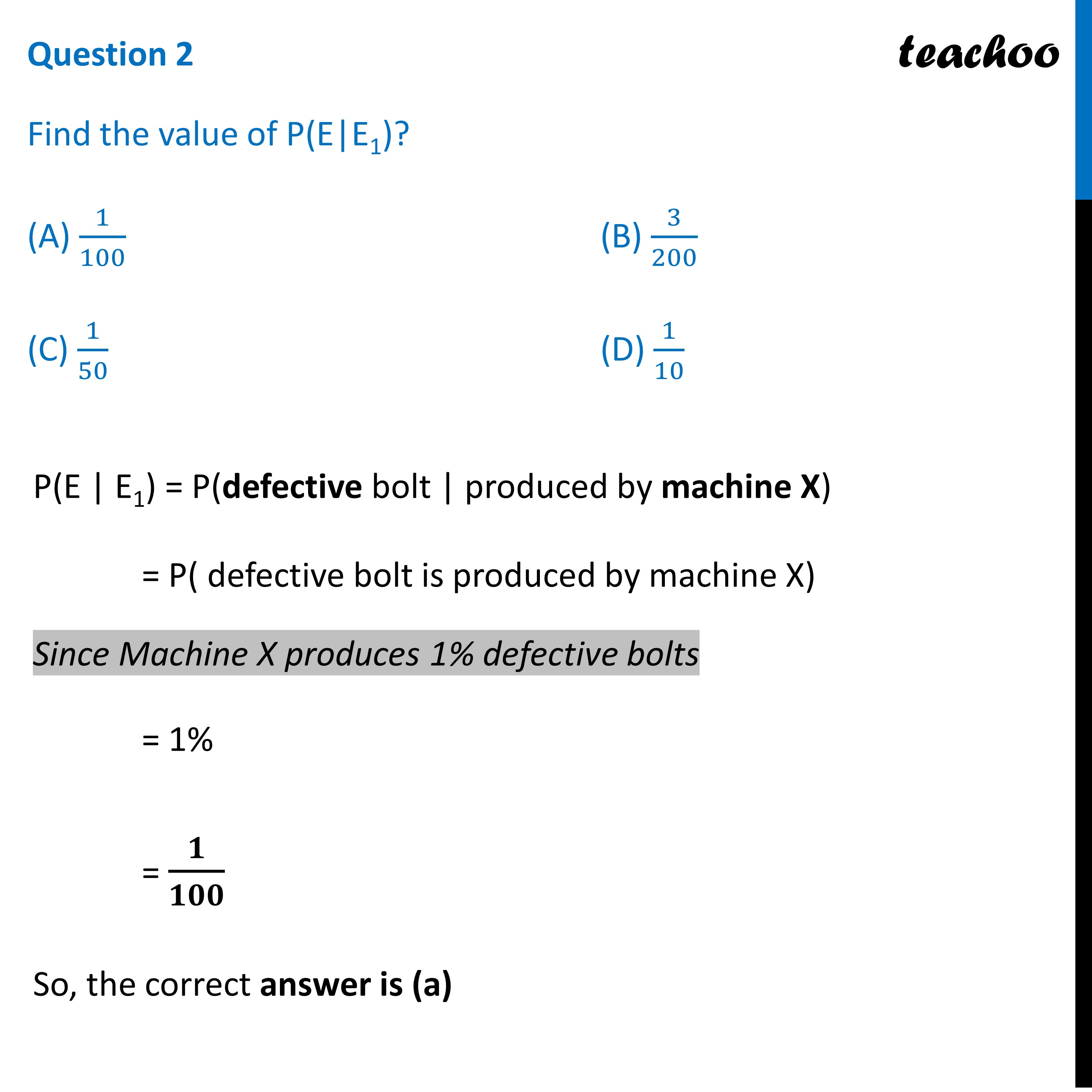## (D) 1/10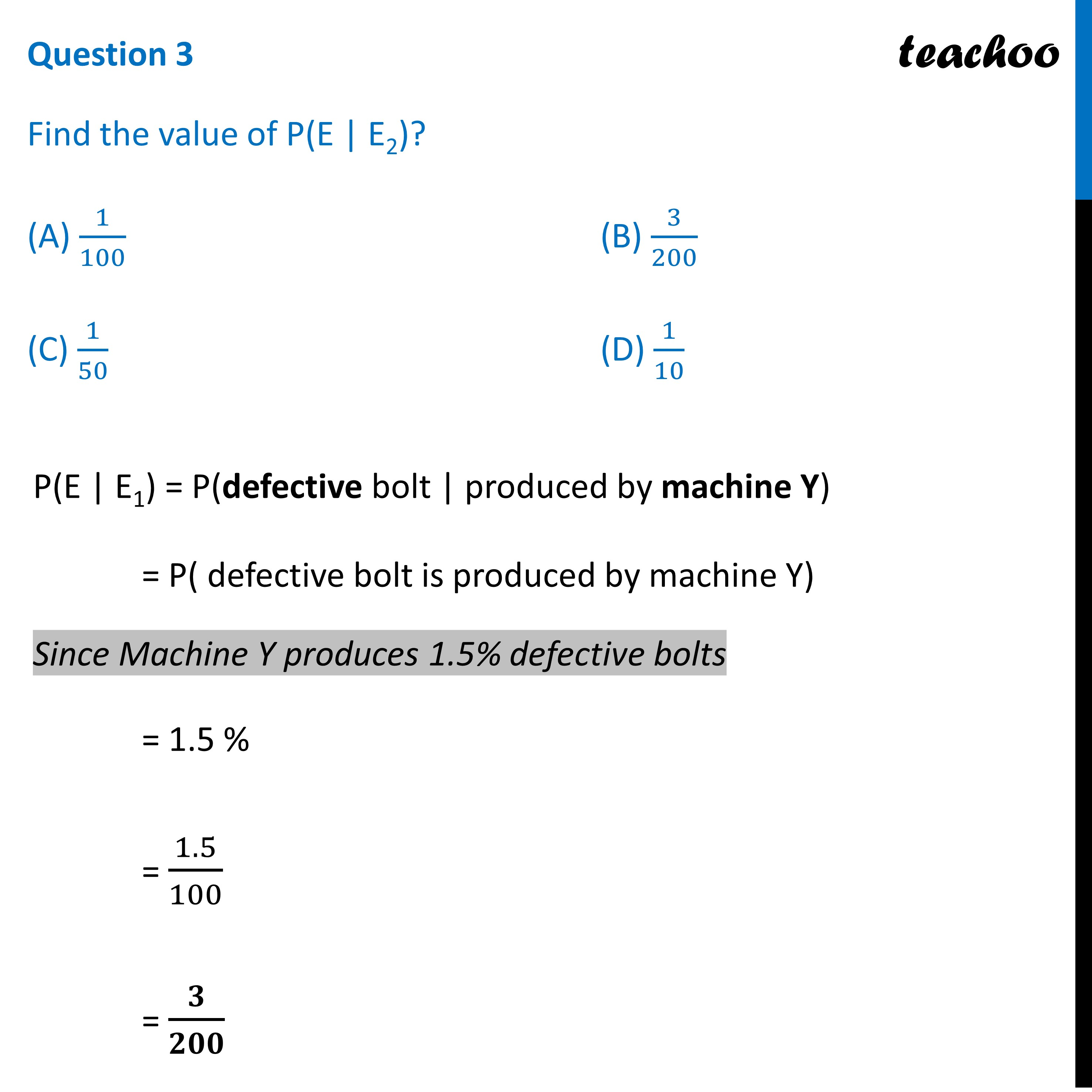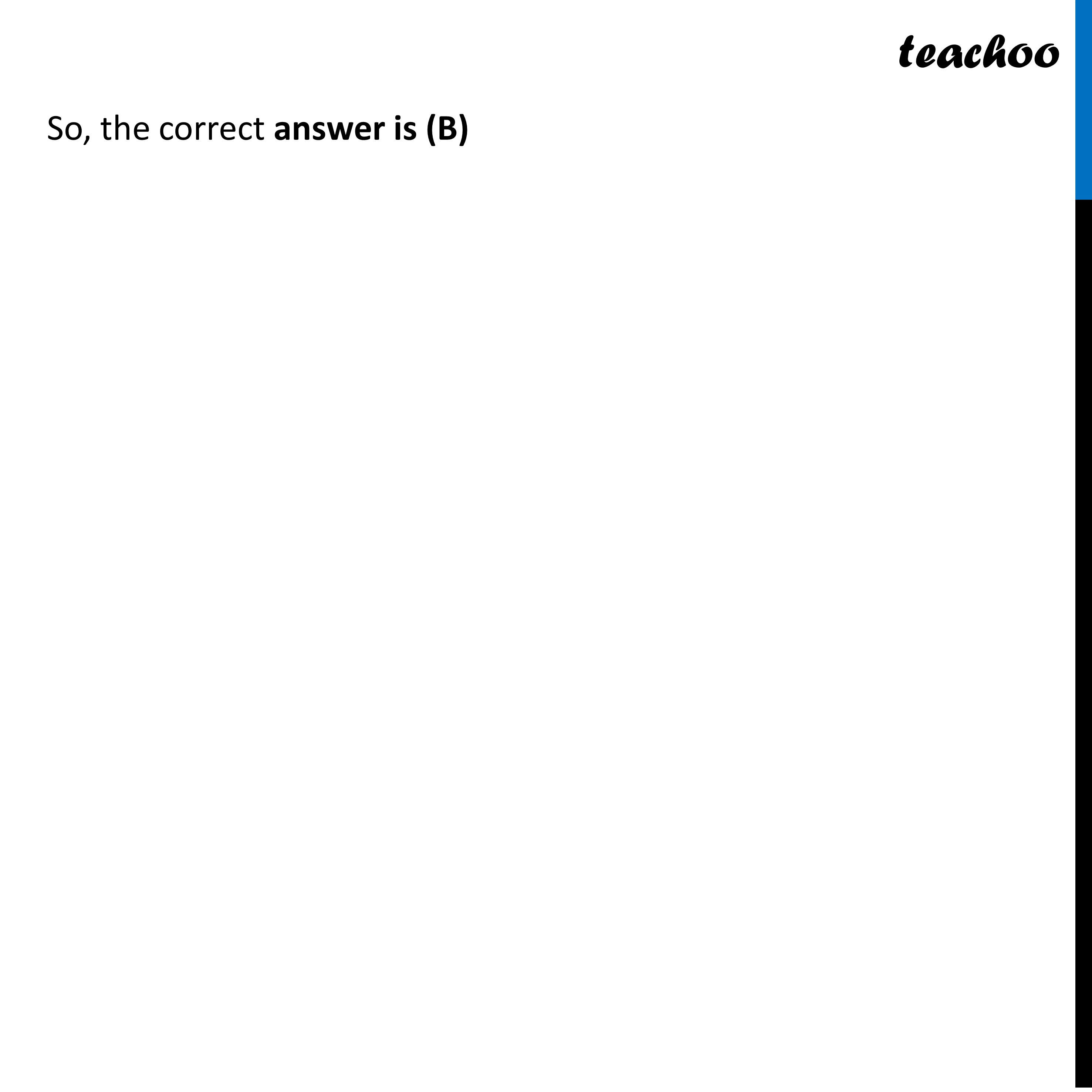## (D) 1/10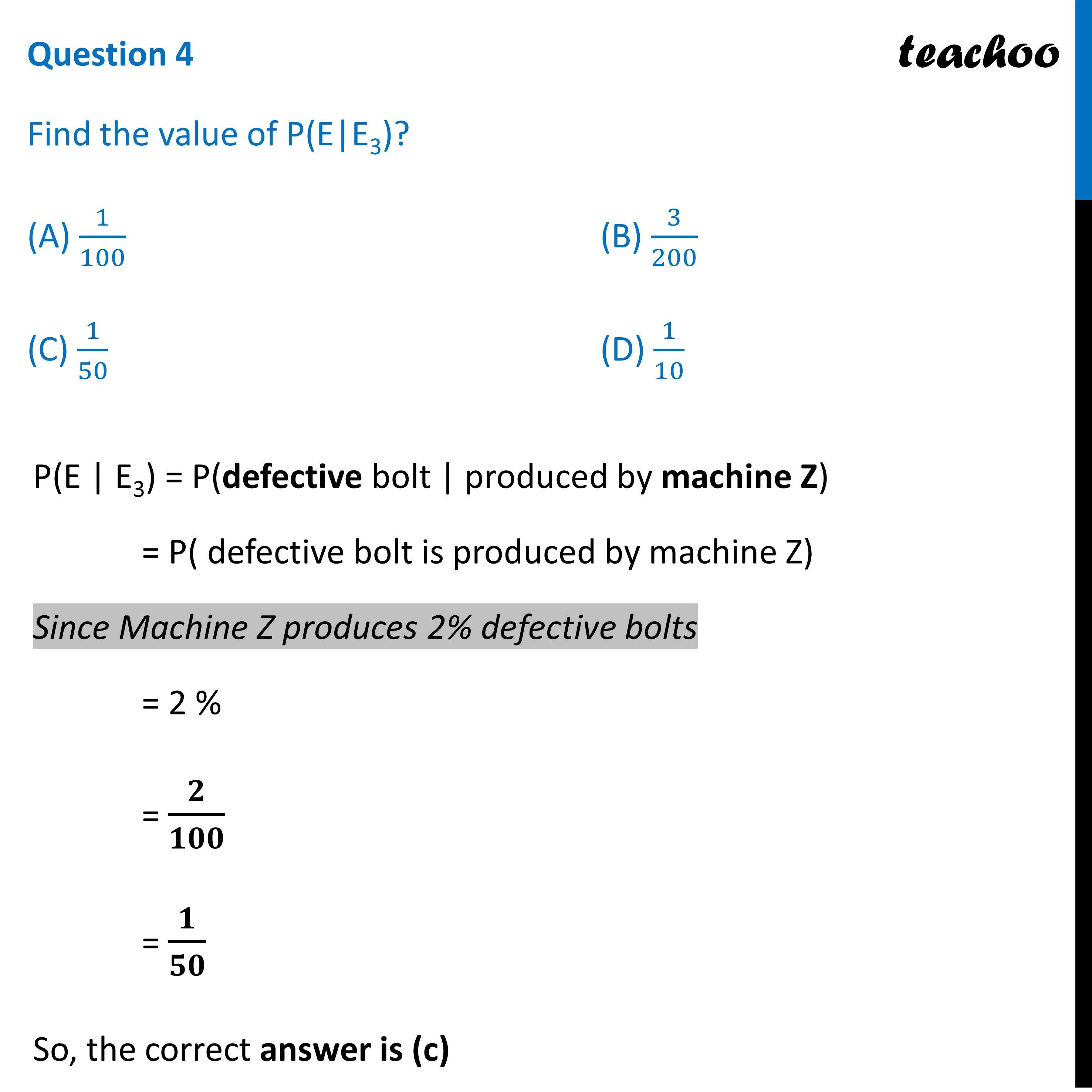## (D) 1/30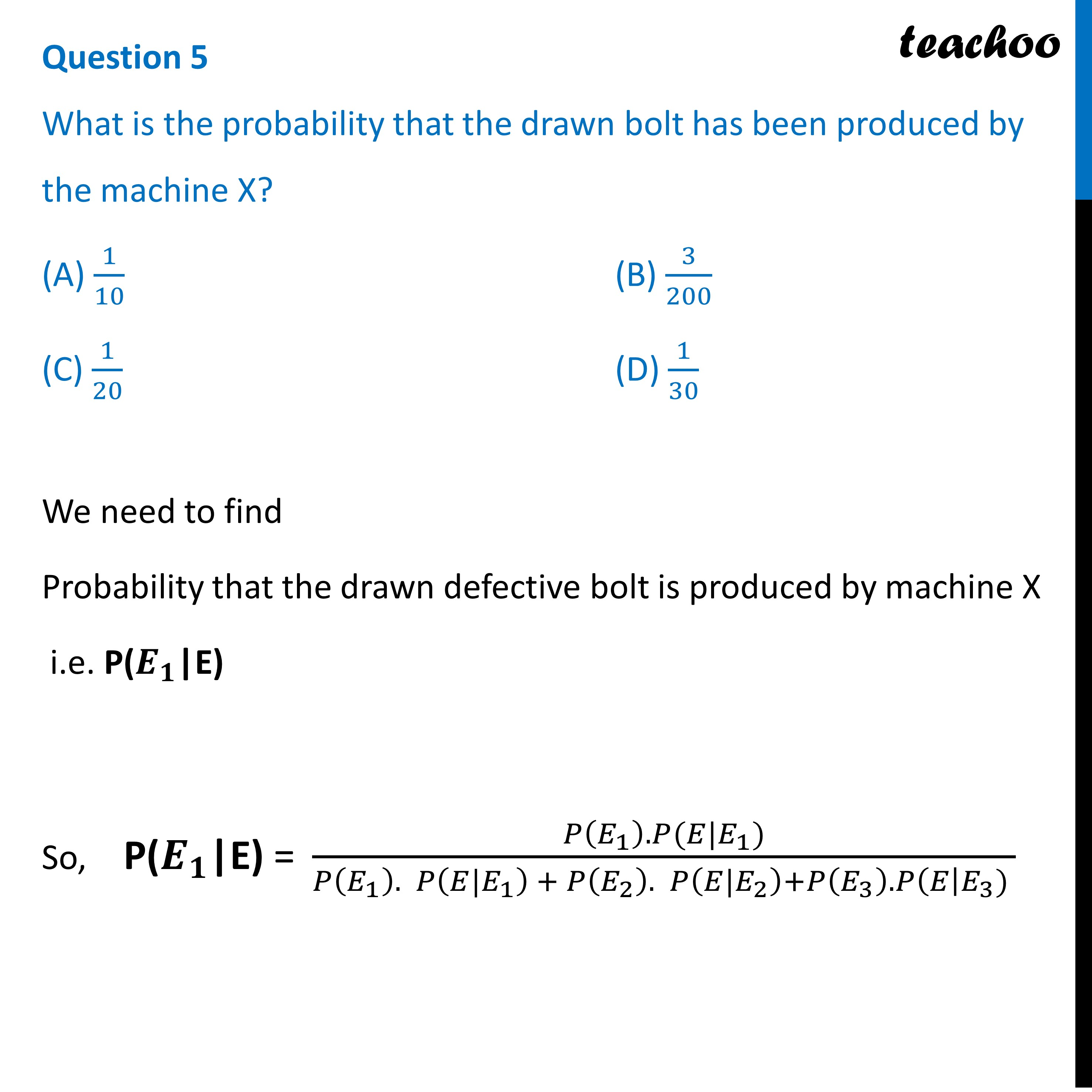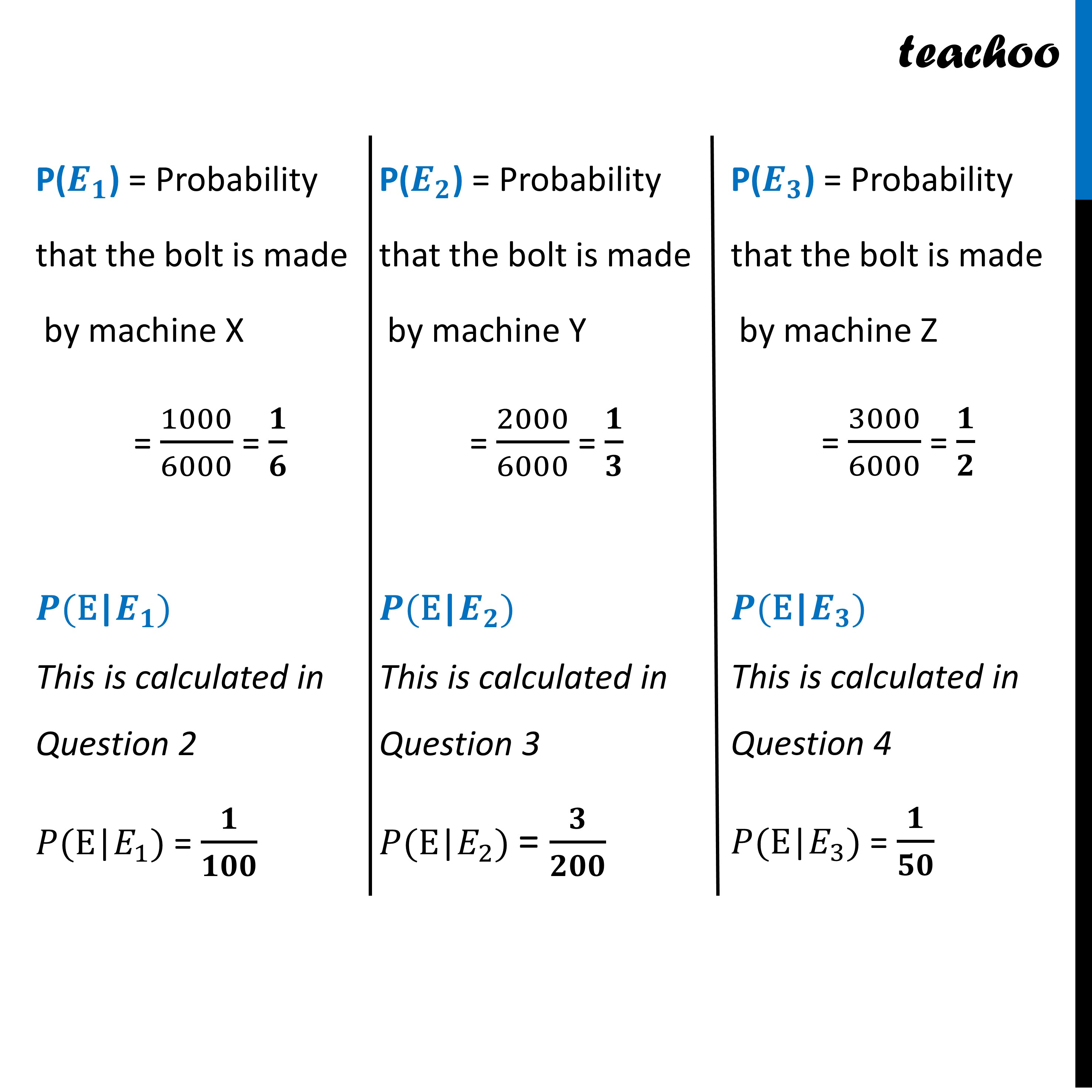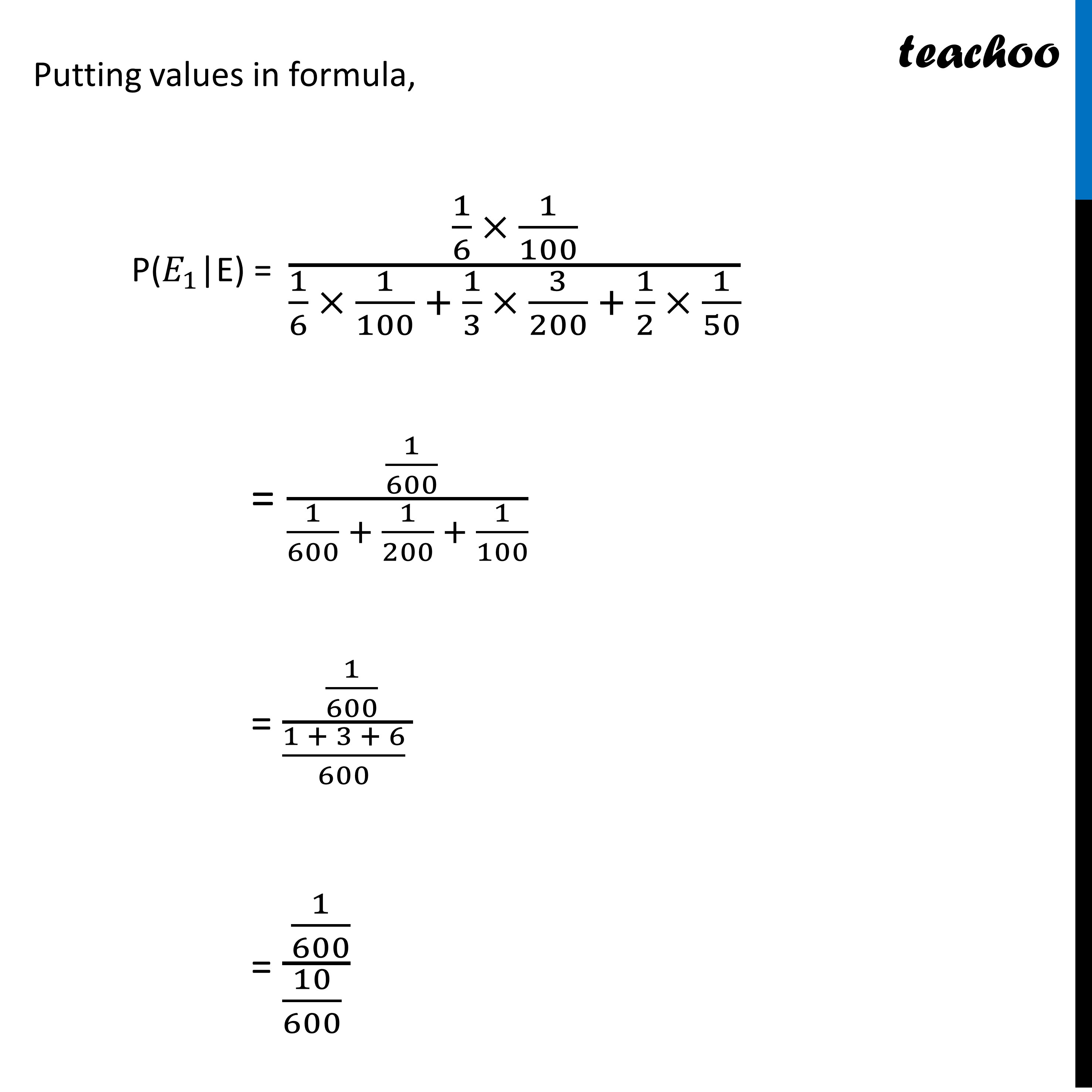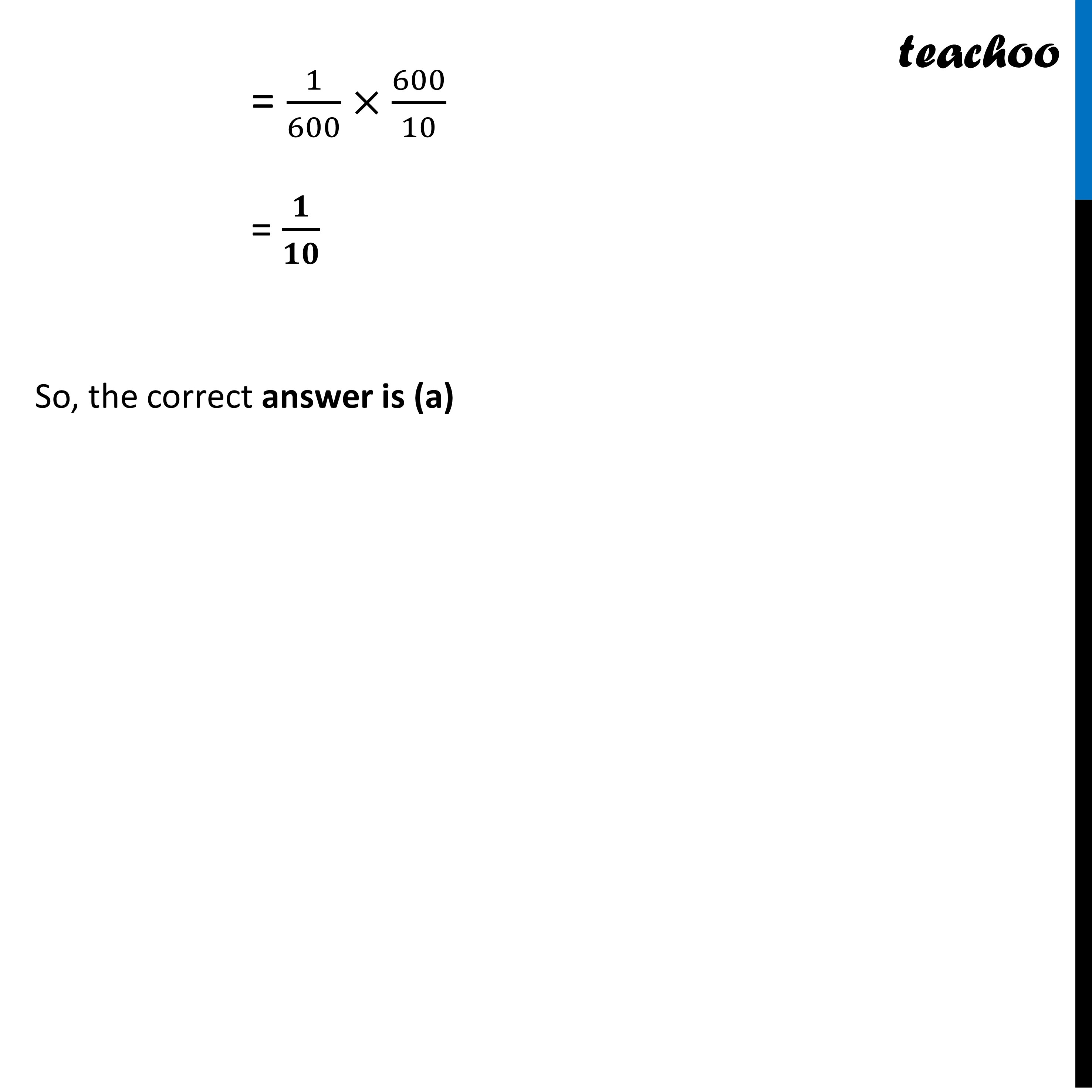Learn in your speed, with individual attention - Teachoo Maths 1-on-1 Class

### Transcript

Question A factory has 3 machines X, Y and Z, producing 1000, 2000 and 3000 bolts per day respectively. The machine X produces 1% defective bolts, Y produces 1.5% defective bolts and Z produces 2% defective bolts. At the end of the day, a bolt is drawn at random and it is found to be defective. Let, E1 = event of drawing a bolt produced by machine X. E2 = event of drawing a bolt produced by machine Y. E3 = event of drawing a bolt produced by machine Z. E = event of drawing a defective bolt. Based on the above information answer the following questions:Question 1 What is the value of P(E2)? (A) 1/6 (B) 1/3 (C) 1/2 (D) 1/4 Now, P(E2) = (𝑁𝑢𝑚𝑏𝑒𝑟 𝑜𝑓 𝑏𝑜𝑙𝑡𝑠 𝑝𝑟𝑜𝑑𝑢𝑐𝑒𝑑 𝑏𝑦 𝑚𝑎𝑐ℎ𝑖𝑛𝑒 𝑌)/(𝑇𝑜𝑡𝑎𝑙 𝑁𝑢𝑚𝑏𝑒𝑟 𝑜𝑓 𝑏𝑜𝑙𝑡𝑠 𝑝𝑟𝑜𝑑𝑢𝑐𝑒𝑑 𝑏𝑦 𝑎𝑙𝑙 3 𝑚𝑎𝑐ℎ𝑖𝑛𝑒𝑠) Number of bolts produced by machine Y = 2000 Total number of defective bolts = 1000 + 2000 + 3000 = 6000 Thus, P(E2) = 2000/6000= 𝟏/𝟑 So, the correct answer is (B) Question 2 Find the value of P(E|E1)? (A) 1/100 (B) 3/200 (C) 1/50 (D) 1/10 P(E | E1) = P(defective bolt | produced by machine X) = P( defective bolt is produced by machine X) Since Machine X produces 1% defective bolts = 1% = 𝟏/𝟏𝟎𝟎 So, the correct answer is (a) Question 3 Find the value of P(E | E2)? (A) 1/100 (B) 3/200 (C) 1/50 (D) 1/10 P(E | E1) = P(defective bolt | produced by machine Y) = P( defective bolt is produced by machine Y) Since Machine Y produces 1.5% defective bolts = 1.5 % = 1.5/100 = 𝟑/𝟐𝟎𝟎 So, the correct answer is (B) Question 4 Find the value of P(E|E3)? (A) 1/100 (B) 3/200 (C) 1/50 (D) 1/10 P(E | E3) = P(defective bolt | produced by machine Z) = P( defective bolt is produced by machine Z) Since Machine Z produces 2% defective bolts = 2 % = 𝟐/𝟏𝟎𝟎 = 𝟏/𝟓𝟎 So, the correct answer is (c) Question 5 What is the probability that the drawn bolt has been produced by the machine X? (A) 1/10 (B) 3/200 (C) 1/20 (D) 1/30 We need to find Probability that the drawn defective bolt is produced by machine X i.e. P(𝑬_𝟏 "|E") So, "P(" 𝑬_𝟏 "|E) = " (𝑃(𝐸_1 ). 𝑃(𝐸|𝐸_1))/(𝑃(𝐸_1 ). 𝑃(𝐸|𝐸_1 ) + 𝑃(𝐸_2 ). 𝑃(𝐸|𝐸_2 )+𝑃(𝐸_3 ). 𝑃(𝐸┤| 𝐸_3) ) "P(" 𝑬_𝟏 ")" = Probability that the bolt is made by machine X = 1000/6000 = 𝟏/𝟔 𝑷("E|" 𝑬_𝟏) This is calculated in Question 2 𝑃("E|" 𝐸_1) = 𝟏/𝟏𝟎𝟎 "P(" 𝑬_𝟐 ")" = Probability that the bolt is made by machine Y = 2000/6000 = 𝟏/𝟑 𝑷("E|" 𝑬_𝟐) This is calculated in Question 3 𝑃("E|" 𝐸_2) = 𝟑/𝟐𝟎𝟎 "P(" 𝑬_𝟑 ")" = Probability that the bolt is made by machine Z = 3000/6000 = 𝟏/𝟐 𝑷("E|" 𝑬_𝟑) This is calculated in Question 4 𝑃("E|" 𝐸_3) = 𝟏/𝟓𝟎 Putting values in formula, "P(" 𝐸_1 "|E) = " (1/6 × 1/100)/(1/6 × 1/100 + 1/3 × 3/200 + 1/2 × 1/50) = ( 1/600)/(1/600 + 1/200 + 1/100) = ( 1/600)/((1 + 3 + 6)/600 ) = ( 1/600)/(10/600 ) Putting values in formula, "P(" 𝐸_1 "|E) = " (1/6 × 1/100)/(1/6 × 1/100 + 1/3 × 3/200 + 1/2 × 1/50) = ( 1/600)/(1/600 + 1/200 + 1/100) = ( 1/600)/((1 + 3 + 6)/600 ) = ( 1/600)/(10/600 ) = 1/600×600/10 = 𝟏/𝟏𝟎 So, the correct answer is (a)